I know the feeling. This topic has a tendency not to fall in our comfort zone. But if we take this up systematically, things can get easy.

What is a differential equation? We have already read about derivatives; the differentiation of a function. So, an equation having (at least one) derivative in it is called a differential equation.

Here are some examples: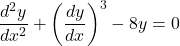Easy enough?

Order: The order of a differential equation is the highest order derivative that appears in a differential equation. Consider the following equation:

Eg:This is a 2nd order differential equation.

## Differential equations are broadly classified into two forms

1. Ordinary differential equations, popularly abbreviated as ODEs: ODEs involve all the derivatives with respect to just one variable in that equation.

Eg: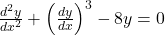Here, all the derivatives (whether first or second order) are with respect to y only.

2. Partial differential equations, popularly abbreviated as PDEs: PDEs involve partial derivatives with respect to several independent variables.

Eg: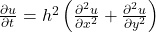In this case, we have partial derivatives with respect to t, x and y.

Notation: Usually, we representas y’. So,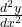would be represented as y’’.

So, Here’s a differential equation (second order)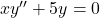.

ODEs can be classified into various categories. In this blog, we are going to take 2 such classifications.

1. Separable: Here’s the usual representation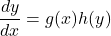As the name suggests if we can separate the functions such that the function of “y” can be written with dy and the function of “x” can be written with dx, it will be categorized as separable.

The Solution would look like:2. Linear: The differential equation of the form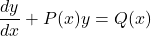is linear equation (first order).

It is solved by first finding “Integrating factor” which is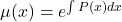.

And the final solution looks like: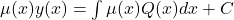.

This list is not exhaustive about the classification of differential equations, but it gives an overview and set ground work for further categories.

Here’s a video in which I have covered various examples.